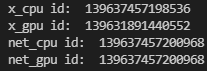# python指定GPU

PyTorch默认使用从0开始的GPU，如果GPU0正在运行程序，需要指定其他GPU。

1. 类似tensorflow指定GPU的方式，使用CUDA_VISIBLE_DEVICES。

1.1 直接终端中设定：

`CUDA_VISIBLE_DEVICES=1 python my_script.py`

1.2 python代码中设定：

```import os
os.environ["CUDA_VISIBLE_DEVICES"] = "2"```

2. 使用函数 set_device

```import torch
torch.cuda.set_device(id)```

• 一种是先调用`t.cuda.set_device(1)`指定使用第二块GPU，后续的`.cuda()`都无需更改，切换GPU只需修改这一行代码。
• 更推荐的方法是设置环境变量`CUDA_VISIBLE_DEVICES`，例如当`export CUDA_VISIBLE_DEVICE=1`（下标是从0开始，1代表第二块GPU），只使用第二块物理GPU，但在程序中这块GPU会被看成是第一块逻辑GPU，因此此时调用`tensor.cuda()`会将Tensor转移至第二块物理GPU。`CUDA_VISIBLE_DEVICES`还可以指定多个GPU，如`export CUDA_VISIBLE_DEVICES=0,2,3`，那么第一、三、四块物理GPU会被映射成第一、二、三块逻辑GPU，`tensor.cuda(1)`会将Tensor转移到第三块物理GPU上
• 设置`CUDA_VISIBLE_DEVICES`有两种方法，一种是在终端命令行中`CUDA_VISIBLE_DEVICES=0,1 python main.py`，一种是在程序中`import os;os.environ["CUDA_VISIBLE_DEVICES"] = "2"`如果使用IPython或者Jupyter notebook，还可以使用`%env CUDA_VISIBLE_DEVICES=1,2`来设置环境变量

3.指定cuda的函数：使用`torch.cuda.device`，来指定默认使用哪一块GPU，或使用`torch.set_default_tensor_type`使程序默认使用GPU，不需要手动调用cuda。

```# 如果未指定使用哪块GPU，默认使用GPU 0
x = t.cuda.FloatTensor(2, 3)
# x.get_device() == 0
y = t.FloatTensor(2, 3).cuda()
# y.get_device() == 0

# 指定默认使用GPU 1
with t.cuda.device(1):
# 在GPU 1上构建tensor
a = t.cuda.FloatTensor(2, 3)

# 将tensor转移至GPU 1
b = t.FloatTensor(2, 3).cuda()
print(a.get_device() == b.get_device() == 1 )

c = a + b
print(c.get_device() == 1)

z = x + y
print(z.get_device() == 0)

# 手动指定使用GPU 0
d = t.randn(2, 3).cuda(0)
print(d.get_device() == 2)```
```t.set_default_tensor_type('torch.cuda.FloatTensor') # 指定默认tensor的类型为GPU上的FloatTensor
a = t.ones(2, 3)
a.is_cuda```

4. tensor.cuda和variable.cuda,都会返回一个新对象，这个新对象的数据已转移至GPU，而之前的tensor/variable的数据还在原来的设备上（CPU）。但是nn.MOdule下的cuda()方法，会将所有数据都迁移至GPU，并返回自己。所以module = module.cuda()和module.cuda()效果相同。通过is_cuda()判断tensor对象是否在cuda上。

```tensor = t.Tensor(3, 4)
# 返回一个新的tensor，保存在第1块GPU上，但原来的tensor并没有改变
tensor.cuda(0)
tensor.is_cuda # False

# 不指定所使用的GPU设备，将默认使用第1块GPU
tensor = tensor.cuda()
tensor.is_cuda # True

module = nn.Linear(3, 4)
module.cuda(device = 1)
module.weight.is_cuda # True```

***后面是常用的方式****

5.判断支持cuda，自动决定是在GPU还是CPU上运行。

```# 在不支持CUDA的机器下，下一步还是在CPU上运行
device = t.device("cuda:0" if t.cuda.is_available() else "cpu")
x = x.to(device)
y = y.to(x.device)
z = x+y```

此外，还可以使用上面的`tensor.cuda()` 的方式将tensor拷贝到gpu上，但是这种方式不太推荐。

6.指定cuda的函数其他方式

```>>> torch.zeros([2, 4], dtype=torch.int32)
tensor([[ 0,  0,  0,  0],
[ 0,  0,  0,  0]], dtype=torch.int32)
>>> cuda0 = torch.device('cuda:0')
>>> torch.ones([2, 4], dtype=torch.float64, device=cuda0)
tensor([[ 1.0000,  1.0000,  1.0000,  1.0000],
[ 1.0000,  1.0000,  1.0000,  1.0000]], dtype=torch.float64, device='cuda:0')```

7.把数据从CPU迁移到GPU时，可以用.cuda()方法，也可以用.to(device)方法。示例如下。

.cuda()方法

``````import torch
import time

t = time.time()
b = torch.rand([1024, 1024, 10])
b = b.cuda()
print('time:{:6.3f}'.format(time.time() - t))  # 输出: time: 0.084``````

.to(device)方法 （推荐）

``````import torch
import time

s = time.time()
device = torch.device('cuda:0' if torch.cuda.is_available() else 'cpu')
a = torch.rand([1024, 1024, 10]).to(device)
print('time:{:6.3f}'.format(time.time()-s)) # 输出: time: 0.087``````

.cuda()方法和.to(device)方法耗时基本差不多。但是.to(device)代码风格更容易后期修改。

8.另外，值得注意到的是，张量在GPU和CPU之间的迁移不是in-place操作（返回新对象），而模型在GPU和CPU之间的迁移是in-place操作（返回原来的对象）。示例如下。

``````import torch
import torch.nn as nn

device = torch.device('cuda:0' if torch.cuda.is_available() else 'cpu')

x_cpu = torch.randn(2, 3)
print('x_cpu id: ', id(x_cpu))

x_gpu = x_cpu.to(device)
print('x_gpu id: ', id(x_gpu))

net_cpu = nn.Linear(32, 10)
print('net_cpu id: ', id(net_cpu))

net_gpu = net_cpu.to(device)
print('net_gpu id: ', id(net_gpu))``````

运行结果：物联沃分享整理
物联沃-IOTWORD物联网 » python指定GPU